Attention !!! We are operating and shipping all Orders. But due to COVID-19 related restrictions, limited staff and reduced working time dispatches may be delayed.
Thanks for your understanding & Support. Be kind and stay safe!!
• 8412906903
• dnatechindia@gmail.com

### Have You Seen

#### STM32F103C8T6 ARM Development Board

STM32F103C8T6 ARM Development Board (Blue Pill)STM32F103C8T6 Dev Board for ARM Microcontroller, STM3..

Rs.932.20 (inc GST)
Rs.790.00 + GST

#### GY-906 MLX90614ESF MODULE

GY-906 MLX90614ESF MODULE GY-906-MLX90614ESF-New-MLX90614-Contactless-Temperature-Sensor-Module-Com..

Rs.1,314.52 (inc GST)
Rs.1,114.00 + GST

# NPN Transistor

## The NPN Transistor

In the previous tutorial we saw that the standard Bipolar Transistor or BJT, comes in two basic forms. An NPN (Negative-Positive-Negative) type and a PNP (Positive-Negative-Positive) type, with the most commonly used transistor type being the NPN Transistor. We also learnt that the transistor junctions can be biased in one of three different ways - Common Base, Common Emitter and Common Collector. In this tutorial we will look more closely at the "Common Emitter" configuration using NPN Transistors with an example of the construction of a NPN transistor along with the transistors current flow characteristics is given below.

### An NPN Transistor Configuration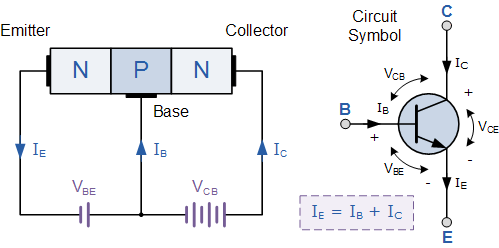(Note: Arrow defines the emitter and conventional current flow, "out" for an NPN transistor.)

The construction and terminal voltages for an NPN transistor are shown above. The voltage between the Base and Emitter ( VBE ), is positive at the Base and negative at the Emitter because for an NPN transistor, the Base terminal is always positive with respect to the Emitter. Also the Collector supply voltage is positive with respect to the Emitter ( VCE ). So for an NPN transistor to conduct the Collector is always more positive with respect to both the Base and the Emitter.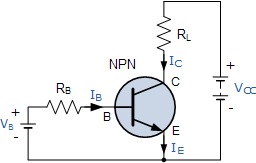Then the voltage sources are connected to an NPN transistor as shown. The Collector is connected to the supply voltage VCC via the load resistor, RL which also acts to limit the maximum current flowing through the device. The Base supply voltage VB is connected to the Base resistor RB, which again is used to limit the maximum Base current.

We know that the transistor is a "current" operated device (Beta model) and that a large current ( Ic ) flows freely through the device between the collector and the emitter terminals when the transistor is switched "fully-ON". However, this only happens when a small biasing current ( Ib ) is flowing into the base terminal of the transistor at the same time thus allowing the Base to act as a sort of current control input.

The transistor current in an NPN transistor is the ratio of these two currents ( Ic/Ib ), called the DC Current Gain of the device and is given the symbol of hfe or nowadays Beta, ( β ). The value of β can be large up to 200 for standard transistors, and it is this large ratio between Ic and Ib that makes the NPN transistor a useful amplifying device when used in its active region as Ib provides the input and Ic provides the output. Note that Beta has no units as it is a ratio.

Also, the current gain of the transistor from the Collector terminal to the Emitter terminal, Ic/Ie, is called Alpha, ( α ), and is a function of the transistor itself (electrons diffusing across the junction). As the emitter current Ie is the product of a very small base current plus a very large collector current, the value of alpha α, is very close to unity, and for a typical low-power signal transistor this value ranges from about 0.950 to 0.999

### α and β Relationship in a NPN Transistor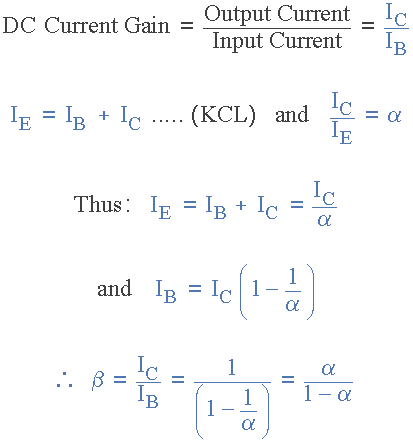By combining the two parameters α and β we can produce two mathematical expressions that gives the relationship between the different currents flowing in the transistor.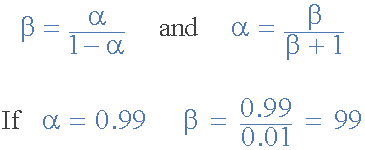The values of Beta vary from about 20 for high current power transistors to well over 1000 for high frequency low power type bipolar transistors. The value of Beta for most standard NPN transistors can be found in the manufactures datasheets but generally range between 50 - 200.

The equation above for Beta can also be re-arranged to make Ic as the subject, and with a zero base current ( Ib = 0 ) the resultant collector current Ic will also be zero, ( β x 0 ). Also when the base current is high the corresponding collector current will also be high resulting in the base current controlling the collector current. One of the most important properties of the Bipolar Junction Transistor is that a small base current can control a much larger collector current. Consider the following example.

### Example No1

An NPN Transistor has a DC current gain, (Beta) value of 200. Calculate the base current Ib required to switch a resistive load of 4mA.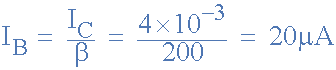Therefore, β = 200, Ic = 4mA and Ib = 20µA.

One other point to remember about NPN Transistors. The collector voltage, ( Vc ) must be greater and positive with respect to the emitter voltage, ( Ve ) to allow current to flow through the transistor between the collector-emitter junctions. Also, there is a voltage drop between the Base and the Emitter terminal of about 0.7v (one diode volt drop) for silicon devices as the input characteristics of an NPN Transistor are of a forward biased diode. Then the base voltage, ( Vbe ) of a NPN transistor must be greater than this 0.7V otherwise the transistor will not conduct with the base current given as.

IB = (VB - VBE)/RB

Where:   Ib is the base current, Vb is the base bias voltage, Vbe is the base-emitter volt drop (0.7v) and Rb is the base input resistor. Increasing Ib, Vbe slowly increases to 0.7V but Ic rises exponentially.

### Example No2

An NPN Transistor has a DC base bias voltage, Vb of 10v and an input base resistor, Rb of 100kΩ. What will be the value of the base current into the transistor.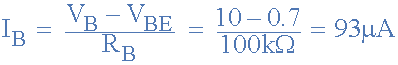Therefore, Ib = 93µA.

## The Common Emitter Configuration

As well as being used as a semiconductor switch to turn load currents "ON" or "OFF" by controlling the Base signal to the transistor in ether its saturation or cut-off regions, NPN Transistors can also be used in its active region to produce a circuit which will amplify any small AC signal applied to its Base terminal with the Emitter grounded. If a suitable DC "biasing" voltage is firstly applied to the transistors Base terminal thus allowing it to always operate within its linear active region, an inverting amplifier circuit called a single stage common emitter amplifier is produced.

One such Common Emitter Amplifier configuration of an NPN transistor is called a Class A Amplifier. A "Class A Amplifier" operation is one where the transistors Base terminal is biased in such a way as to forward bias the Base-emitter junction. The result is that the transistor is always operating halfway between its cut-off and saturation regions, thereby allowing the transistor amplifier to accurately reproduce the positive and negative halves of any AC input signal superimposed upon this DC biasing voltage. Without this "Bias Voltage" only one half of the input waveform would be amplified. This common emitter amplifier configuration using an NPN transistor has many applications but is commonly used in audio circuits such as pre-amplifier and power amplifier stages.

With reference to the common emitter configuration shown below, a family of curves known as the Output Characteristics Curves, relates the output collector current, (Ic) to the collector voltage, (Vce) when different values of Base current, (Ib) are applied to the transistor for transistors with the same β value. A DC "Load Line" can also be drawn onto the output characteristics curves to show all the possible operating points when different values of base current are applied. It is necessary to set the initial value of Vce correctly to allow the output voltage to vary both up and down when amplifying AC input signals and this is called setting the operating point or Quiescent Point, Q-point for short and this is shown below.

### Single Stage Common Emitter Amplifier Circuit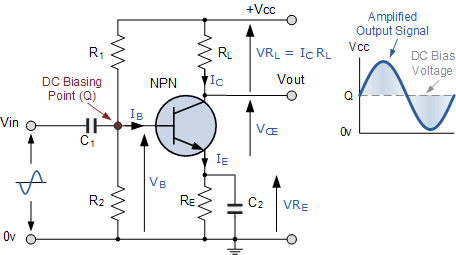### Output Characteristics Curves of a Typical Bipolar Transistor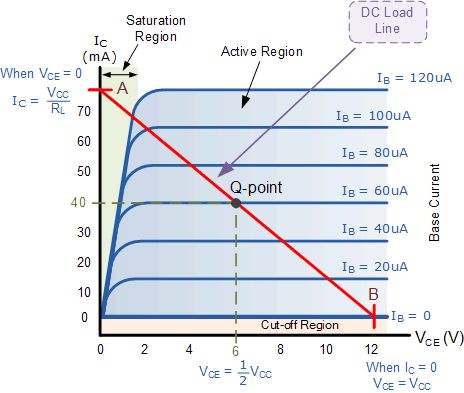The most important factor to notice is the effect of Vce upon the collector current Ic when Vce is greater than about 1.0 volts. We can see that Ic is largely unaffected by changes in Vce above this value and instead it is almost entirely controlled by the base current, Ib. When this happens we can say then that the output circuit represents that of a "Constant Current Source". It can also be seen from the common emitter circuit above that the emitter current Ie is the sum of the collector current, Ic and the base current, Ib, added together so we can also say that Ie = Ic + Ib for the common emitter (CE) configuration.

By using the output characteristics curves in our example above and also Ohm´s Law, the current flowing through the load resistor, (RL), is equal to the collector current, Ic entering the transistor which inturn corresponds to the supply voltage, (Vcc) minus the voltage drop between the collector and the emitter terminals, (Vce) and is given as:

Collector Current,  IC = (VCC - VCE)/RL

Also, a straight line representing the Dynamic Load Line of the transistor can be drawn directly onto the graph of curves above from the point of "Saturation" ( A ) when Vce = 0 to the point of "Cut-off" ( B ) when Ic = 0 thus giving us the "Operating" or Q-point of the transistor. These two points are joined together by a straight line and any position along this straight line represents the "Active Region" of the transistor. The actual position of the load line on the characteristics curves can be calculated as follows:

When, ( VCE = 0 ),  IC = (VCC - 0 )/RL , IC = VCC / RL

When, ( IC = 0 ),  0 = (VCC - VCE)/RL, VCC = VCE

Then, the collector or output characteristics curves for Common Emitter NPN Transistors can be used to predict the Collector current, Ic, when given Vce and the Base current, Ib. A Load Line can also be constructed onto the curves to determine a suitable Operating or Q-point which can be set by adjustment of the base current. The slope of this load line is equal to the reciprocal of the load resistance which is given as: -1/RL

Then we can define a NPN Transistor as being normally "OFF" but a small input current and a small positive voltage at its Base (B) relative to its Emitter (E) will turn it "ON" allowing a much large Collector-Emitter current to flow. NPN transistors conduct when Vc is much greater than Ve.

In the next tutorial about Bipolar Transistors, we will look at the opposite or complementary form of the NPN Transistor called the PNP Transistor and show that the PNP Transistor has very similar characteristics to their NPN transistor except that the polarities (or biasing) of the current and voltage directions are reversed.

Reproduced with permission from Wayne Storr
http://www.electronics-tutorials.ws/transistor/tran_2.htmlWebsite: